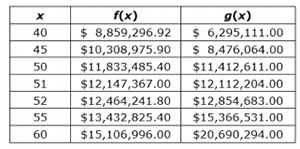# Sample Test Item PreviewExport Print
General Information
Related Benchmark: MAFS.912.F-LE.1.3
Reporting Category: Functions: Linear, Quadratic, & Exponential Models
Type: OR: Open Response
Difficulty: N/A
Question:

The function f(x) models the value of goods that are imported into the United States, where x is the number of years since 1990. The function g(x) models the value of goods that are exported from the United States.If f(x) and g(x) continue to model the importing and exporting of goods, then sometime in 2041, which is 51 years after 1990, f(x)=g(x).

Determine which function is exponential. Use the table of values to justify your answer.